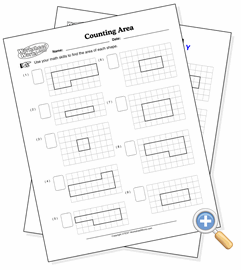# Counting AreaUse geometry with basic math to compute total areas of shapes

This worksheet introduces the concept of shape area without forcing the student to actually make any computations. If they want to, they can simply count the number of squares that the shape makes up. If they already understand multiplication, they will quickly realize that they can compute the area much faster by using that skill. At higher difficulty levels, parts of the shapes often have sides that are not at clean ninety degree angles. You may want to explain how they can find the answer by first treating the area as a rectangle, then dividing the result by two. In all cases, the area of shapes will come out to be exact integers, so no knowledge of fractions is necessary for this exercise.